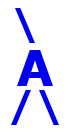## AIRES library reference: olv2slant.

```c
c     Routine directly callable from C/C++ (it could be necessary to
c     append an underscore, "_", to its name depending on the C/C++
c     compiler used).
c
c
subroutine olv2slant(nobslev, olxv, x0, zendis, zen1, zen2,
+                     zcground, olxs)
c
c     Evaluating the slant depth of a set of observing levels. The slant
c     depths are calculated along an axis starting at altitude zcground,
c     for the "segment" that ends at vertical depth x0 (x0 = 0 is the
c     top of the atmosphere). The integer variable "zendis" allows to
c     select among fixed, sin and sin-cos zenith angle distributions.
c
c     Written by: S. J. Sciutto, Fermilab 1999.
c
c
c     Arguments:
c     =========
c
c     nobslev......... (input, integer) The number of observing
c                      levels.
c     olxv............ (input, double precision, array(nobslev))
c                      Vertical depths (g/cm^2) of the corresponding
c                      observing levels.
c     x0.............. (input, double precision) Vertical depth of
c                      the paths' end points (g/cm2). If x0 is zero
c                      then the paths are evaluated up to the top of
c                      the atmosphere.
c     zendis.......... (input, integer) Zenith angle distribution
c                      switch: 0 - fixed zenith angle, 1 - sin
c                      distribution, 2 - sin cos distribution.
c     zen1, zen2...... (input, double precision) Minimum and maximum
c                      zenith angles (degrees). If zendis is 0, then
c                      zen2 is not used and zen1 gives the
c                      corresponding fixed zenith angle.
c     zcground........ (input, double precision) Central altitude of
c                      the ground level, which defines the intersection
c                      between the slanted axis and the z-axis.
c     olxv............ (output, double precision, array(nobslev))
c                      Slant depths (g/cm^2) of the corresponding
c                      observing levels.
c
c
c<--->
```

 These pages are maintained by Sergio J. Sciutto Back to AIRES library index Back to AIRES home